# 光Hello?

## 如何思考传统算法问题——以 Union-Find 算法为例

2019.07.05

“怎样系统地思考一个算法问题？”
“为什么我只会解决见过的算法题，对陌生的算法题不知道怎样着手？”


《算法（第4版）》在第一章就解决了这个新手的困扰，介绍思考任意传统算法问题的科学思路。本文做一个梳理，通过Union-find算法作为例子，总结面对一个传统算法场景的思考方向。

### 总体思路

 定义基本操作API -> 定义测试用例 -> 实现一个简单方案 -> 分析算法效率 -> 优化迭代


## 以 Union-Find 问题为例：

##### 什么是 Union-Find 问题？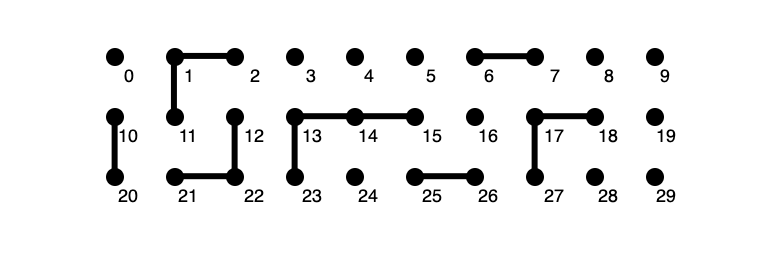1. 输入一串数字，每两个数字为一组，比如 tinyUF.txt：

» cat tinyUF.txt
15
4 3
3 8
6 5
9 4
2 1
8 9
5 0
7 2
6 1
1 0
6 7


2. 从第二行开始每次读2个数字为一组，判断这两个数字代表的点是否连接。（这里定义的连接可以传递，比如a连接b，b连接c，那么a与c也连接。）
3. 如果不连接，实现算法让其连接；如果连接，返回true，然后继续读下一组数。

### Step 1. 定义API

（据我观察，工作中遇到的很多问题，都是因为没把问题本身想清楚，当你明确了问题之后，问题自然解决了。）

##### 定义 API 就是抽象问题的过程
• 用 int[] 表示所有点的集合。
这相当于把每个点抽象成数组里的一个元素，元素的角标用来确定这个点的位置，元素的值代表这个点的某种信息，比如所在分量的标识。
• 把互相连接的点的集合称为分量
• 接下来定义解决这个问题的类：
class UF{
//    所有点的集合
private int[] id;
//    分量数量
private int count;
//    初始化
public UF(int N) { }
//    查找分量标识符
public int find(int p) { }
//    两个触点是否连接，也就是是否在同一个分量
public boolean connected(int p, int q) { }
//    连接两个触点
public void union(int p, int q){ }
public int count() { }
}


//根据角标找到这个点的值
public int find(int p) {}
//连接两个点，参数为角标
public void union(int p, int q) {}


public abstract class UF {
//    所有点的集合
private int[] id;
//    分量数量
private int count;
//    初始化
public UF(int N) {
count = N;
id = new int[N];
for (int i = 0; i < N; i++) {
id[i] = i;
}
}
//    查找分量标识符
abstract int find(int p);
//    两个点是否连接，也就是是否在同一个分量
public boolean connected(int p, int q) {
return find(p) == find(q);
}
//todo    连接两个点，需要在接下来实现
abstract public void union(int p, int q);
public int count() {
return count;
}
}


### Step 2. 定义测试用例

public class UFQuickFind extends UF {
// 具体需要实现的方法
public UFQuickFind(int N) {
super(N);
}
//    查找分量标识符
public int find(int p) {
return id[p];
}
//具体需要实现的方法
public void union(int p, int q) {}
//测试用例
public static void main(String[] args) {
Scanner scanner = new Scanner(System.in);
int count = scanner.nextInt();
UFQuickFind qf = new UFQuickFind(count);
while (scanner.hasNextInt()) {
int p = scanner.nextInt();
int q = scanner.nextInt();
//            判断是否连接
if (qf.connected(p, q)) {
continue;
}
//            如果没连接，则归并分量
qf.union(p, q);
//            打印连接
System.out.println(p + " " + q);
}
System.out.println(qf.count() + "components");
}
}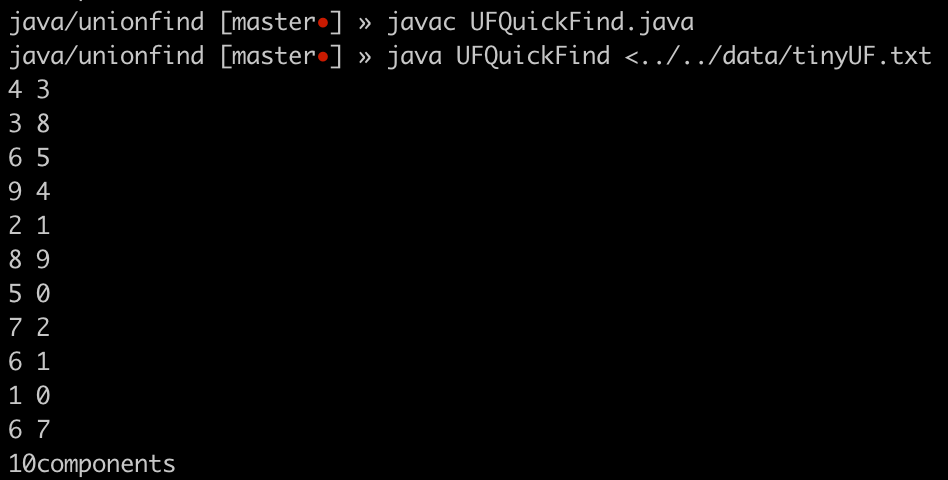### Step 3. 简单实现：quick-find 算法

id == id


##### 实现 find() 方法：

public int find(int p) {
return id[p];
}

##### 实现 union() 方法：

union() 方法用来连接两个点，只需要遍历每个元素，把一个点所属分量合并到另一个点所属的分量。

    public void union(int p, int q) {
//        找p和q各自所属分量
int pGroup = find(p);
int qGroup = find(q);
if (pGroup == qGroup) {
return;
}
//        遍历所有元素，把与q同一个分量的元素放到p所属的分量
for (int t = 0; t < id.length; t++) {
if (id[t] == qGroup) {
id[t] = pGroup;
}
}
count--;
}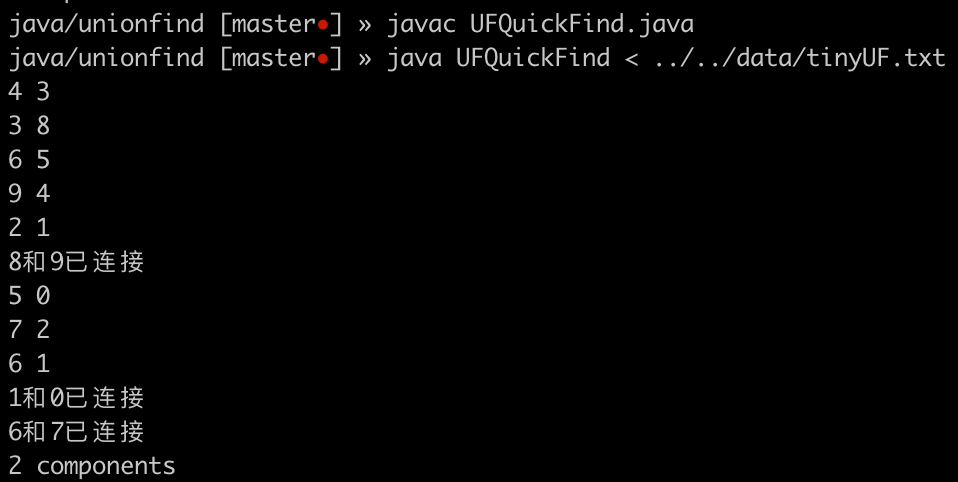### Step 4. 分析效率

• find() 时间复杂度 O(1)
• union() 时间复杂度 O(N)，N就是数组长度
• 如果想用 quick-find 算法把所有数据连通，最后只剩下一个分量，最坏情况需要调用 N-1次 union()，那么很明显，这种情况下，时间复杂度 O(N^2)。

### Step 5. 优化：quick-union 算法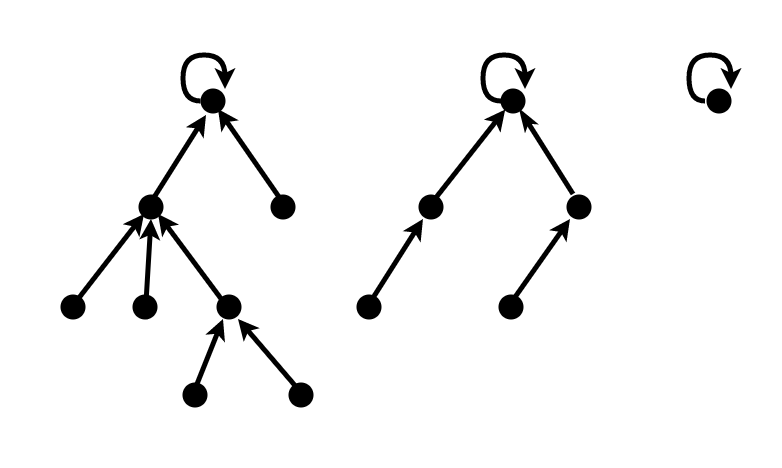##### 实现 find()

public int find(int p) {
int pVal = id[p];
if (pVal == p) {
return p;
}
return find(pVal);
}


public int find(int p) {
while (p != id[p]) {
p = id[p];
}
return p;
}

##### 实现 union()

public void union(int p, int q) {
int pRoot = find(p);
int qRoot = find(q);
if (pRoot == qRoot) {
return;
}
id[pRoot] = qRoot;
count--;
return;
}


## 重复 Step 4 - Step 5 (分析效率 -> 优化算法 -> 分析效率 -> 优化算法……)

### 分析 quick-union 效率

##### find() 方法时间复杂度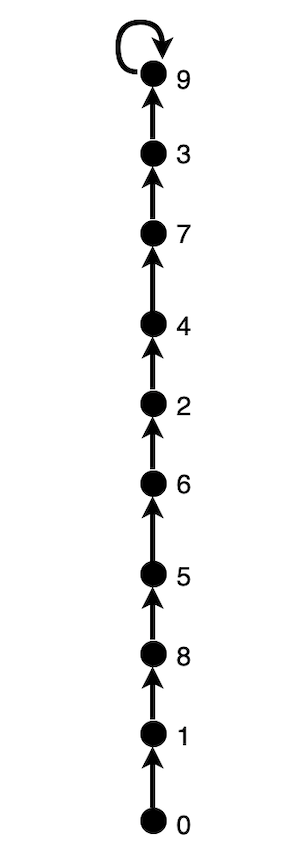0-1 0-2 0-3 0-4 0-5 0-6 0-7 0-8 0-9


### 继续优化 quick-union 算法：weighted-quick-union 算法

private int[] height;
//构造方法中初始化 height
public UFWeightedQuickUnion(int N) {
count = N;
id = new int[N];
for (int i = 0; i < N; i++) {
id[i] = i;
}
height = new int[N];
for (int i = 0; i < N; i++) {
height[i] = i;
}
}


union() 方法：

void union(int p, int q) {
int pRoot = find(p);
int qRoot = find(q);
if (pRoot == qRoot) {
return;
}
int pH = height[pRoot];
int qH = height[qRoot];
if (pH > qH) {
id[qRoot] = pRoot;
height[pRoot]++;
} else {
id[pRoot] = qRoot;
height[qRoot]++;
}
count--;
return;
}


### 分析 weighted-quick-union 算法

• find() 方法复杂度 O(lgN)
• union() 方法复杂度 O(lgN)
• 如果要把所有点合到一个分量，最坏情况的复杂度 O((N -1) * lgN) ~ O(N * lgN)

### 最优算法：路径压缩 weighted-quick-union 算法

int find(int p) {
int start = p;
//        让 p 取到根节点的值
while (p != id[p]) {
p = id[p];
}
//        重新遍历，把路径上的节点都指向根节点
while (start != p) {
int temp = start;
start = id[start];
id[temp] = p;
}
return p;
}